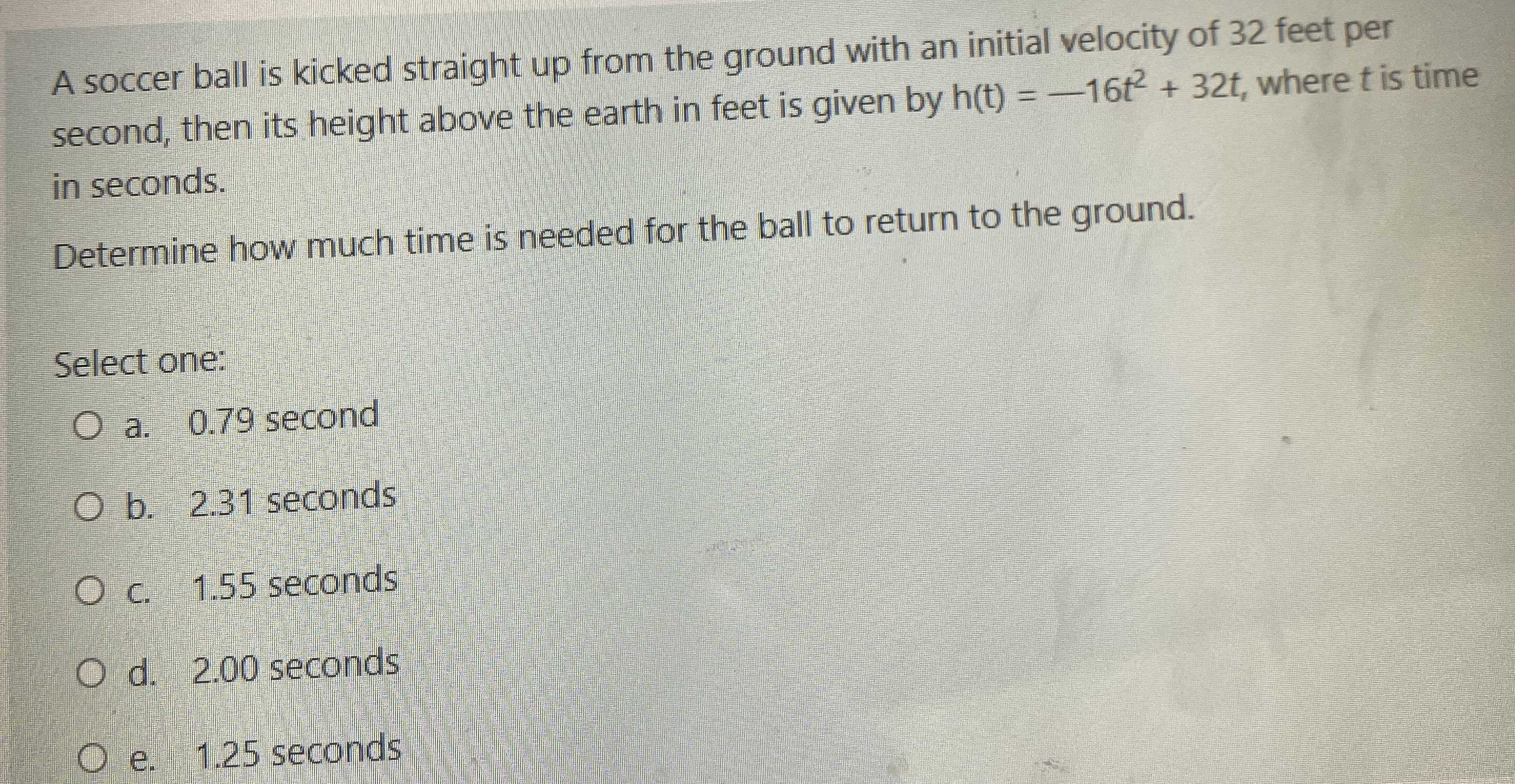### Still have math questions?

Algebra
QuestionA soccer ball is kicked straight up from the ground with an initial velocity of $$32$$ feet per second, then its height above the earth in feet is given by $$h ( t ) = - 16 t ^ { 2 } + 32 t$$ , where $$t$$ is time in seconds.

Determine how much time is needed for the ball to return to the ground.

Select one:

a. $$0.79$$ second

b. $$2.31$$ seconds

c. $$1.55$$ seconds

d. $$2.00$$ seconds

e. $$1.25$$ seconds